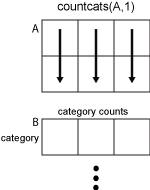# countcats

Count occurrences of categorical array elements by category

## Syntax

``B = countcats(A)``
``B = countcats(A,dim)``

## Description

example

````B = countcats(A)` returns the number of elements in each category of the categorical array, `A`. If `A` is a vector, then `countcats` returns the number of elements in each category.If `A` is a matrix, then `countcats` treats the columns of `A` as vectors and returns the category counts for each column of `A`.If `A` is a multidimensional array, then `countcats` acts along the first array dimension whose size does not equal `1`. ```

example

````B = countcats(A,dim)` returns the category counts along dimension `dim`.For example, you can return the category counts of each row in a categorical array using `countcats(A,2)`.```

## Examples

collapse all

Create a 1-by-5 categorical vector.

`A = categorical({'plane' 'car' 'train' 'car' 'plane'})`
```A = 1x5 categorical plane car train car plane ```

`A` has three categories. To list the categories and their order, use the `categories` function. Every element of `A` belongs to one of the categories listed by the call to `categories`.

`categories(A)`
```ans = 3x1 cell {'car' } {'plane'} {'train'} ```

Find the number of elements in each category of `A`.

`B = countcats(A)`
```B = 1×3 2 2 1 ```

The first element in `B` corresponds to the first category of `A`, which is `car`. The second element in `B` corresponds to the second category of `A`, which is `plane`. The third element of `B` corresponds to the third category of `A`, which is `train`.

Since `A` is a row vector, `countcats` returns a row vector.

Create a 3-by-2 categorical array, `A`, from a numeric array.

```valueset = 1:3; catnames = {'red' 'green' 'blue'}; A = categorical([1 3; 2 1; 3 1],valueset,catnames)```
```A = 3x2 categorical red blue green red blue red ```

`A` has three categories, `red`, `green`, and `blue`.

Find the category counts of each column in `A`.

`B = countcats(A)`
```B = 3×2 1 2 1 0 1 1 ```

The first row of `B` corresponds to the first category of `A`. The value, `red`, occurs once in the first column of `A` and twice in the second column.

The second row of `B` corresponds to the second category of `A`. The value, `green`, occurs once in the first column of `A`, and it does not occur in the second column.

The third row of `B` corresponds to the third category of `A`. The value, `blue`, occurs once in the first column of `A` and once in the second column.

Create a 3-by-2 categorical array, `A`, from a numeric array.

```valueset = 1:3; catnames = {'red' 'green' 'blue'}; A = categorical([1 3; 2 1; 3 1],valueset,catnames)```
```A = 3x2 categorical red blue green red blue red ```

`A` has three categories, `red`, `green`, and `blue`.

Find the category counts of `A` along the second dimension.

`B = countcats(A,2)`
```B = 3×3 1 0 1 1 1 0 1 0 1 ```

The first column of `B` corresponds to the first category of `A`. The value, `red`, occurs once in the first row of `A`, once in the second row, and once in the third row.

The second column of `B` corresponds to the second category of `A`. The value, `green`, occurs in only one element. It occurs in the second row of `A`.

The third column of `B` corresponds to the third category of `A`. The value, `blue`, occurs once in the first row of `A` and once in the third row.

Create a 6-by-1 categorical array, `A`, from a numeric array.

```valueset = 1:3; catnames = {'red' 'green' 'blue'}; A = categorical([1;3;2;1;3;1],valueset,catnames)```
```A = 6x1 categorical red blue green red blue red ```

Remove the `blue` category.

`A = removecats(A,'blue')`
```A = 6x1 categorical red <undefined> green red <undefined> red ```

`A` has two categories, `red` and `green`. Elements of `A` that were from the `blue` category are now undefined.

Find the number of elements in each category of `A`.

`B = countcats(A)`
```B = 2×1 3 1 ```

The first element in `B` corresponds to the first category of `A`. The value, `red`, occurs three times in `A`.

The second element in `B` corresponds to the second category of `A`. The value, `green`, occurs once in `A`.

`countcats` does not return any information on undefined elements.

Use the `summary` function to view the number of undefined elements in addition to the number of elements in each category of `A`.

`summary(A)`
``` red 3 green 1 <undefined> 2 ```

## Input Arguments

collapse all

Categorical array, specified as a vector, matrix, or multidimensional array.

Dimension to operate along, specified as a positive integer scalar. If you do not specify the dimension, then the default is the first array dimension of size greater than 1.

Consider a two-dimensional categorical array, `A`.

If `dim = 1`, then `countcats(A,1)` returns the category counts for each column of `A`.If `dim = 2`, then `countcats(A,2)` returns the category counts of each row of `A`.If `dim` is greater than `ndims(A)`, then `countcats(A)` returns an array the same size as `A` for each category. `countcats` returns `1` for elements in the corresponding category and `0` otherwise.

## Tips

• To find the number of undefined elements in a categorical array, `A`, you must use `summary` or `isundefined`.

## Version History

Introduced in R2013b